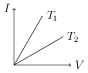# Ohm's Law

## Problems from IIT JEE

Problem (IIT JEE 1985):The current-voltage graphs for a given metallic wire at two different temperatures $T_1$ and $T_2$ are shown in figure. The temperature $T_2$ is greater than $T_1$. (True/False)?

Solution: Let $R_1$ and $R_2$ be resistances of the metallic wire at temperature $T_1$ and $T_2$, respectively. Ohm's law, $V=IR$, gives slope of the $I\text{-}V$ graph as $\mathrm{d}I/\mathrm{d}V=1/R$. In given graph, slope at $T_1$ is greater than slope at $T_2$ i.e., $1/R_1 > 1/R_2$ or $R_1 < R_2$. The resistance of the metallic wire varies with temperature as, \begin{align} \label{ubb:eqn:1} R_1=R_0(1+\alpha(T_1-T_0),\\ \label{ubb:eqn:2} R_2=R_0(1+\alpha(T_2-T_0), \end{align} where $\alpha > 0$ is thermal coefficient of resistance and $R_0$ is resistance at temperature $T_0$. Substitute $R_1$ and $R_2$ from above equations into the inequality $R_1 < R_2$ to get $T_1 < T_2$.